Question

# A 400 g particle moving along the z-axis experiences the force shown in (Figure 1). The...

A 400 g particle moving along the z-axis experiences the force shown in (Figure 1). The particle's velocity is 2.0m/s at x = 0m. You may want to review (Pages 214 - 218)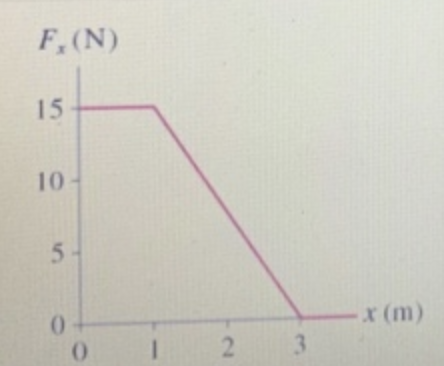Part A

What is its velocity at x= 3 m?

Express your answer to two significant figures and include the appropriate units.

Mass of particle = 400 g = 0.4 kg

Force applied = 15 N from x = 0 to x = 1.

Now,

F = m * a

=> a = F/m = 15/0.4 = 37.5 m/sec^2 .

Let  t be the time taken to move from x = 0 to x = 1.

So,

As per formula : s = u t + 1/2 a t^2

=> 1 = 2.0 t + 1/2 (37.5) * t^2

=> 18.75 t^2 + 2.0 t - 1 = 0

=> t = - 2/ (2*18.75)  +/- sqrt(2^2 - 4*18.75*(-1)) / (2*18.75)

=> t = - 0.29 , 0.1837 secs.

Discard the negative value which is meaningless.

=> t = 0.1837 sec.

Hence, velocity at x = 1, V1 = u + a t = 2 + 37.5 * 0.1837 = 8.89 m/sec

From x = 1 to x = 3, F is decreasing at a constant rate. So, we can take average F as acting during this interval.

Hence, F’ = (15 + 0)/2 = 7.5 N in the interval (1, 3).

So, acceleration a’ in that interval = F’/a’ = 7.5/0.4 = 18.75 m/sec^2

For moving from x = 1 to x = 3, if time taken is t’,

then :

s = V1 t’ + 1/2 a’ t’^2

=> 2 = 8.89 * t’ + 1/2 * 18.75 t’^2

=> 9.375 t’^2 + 8.89 t’ - 2 = 0

=> t’ = - 8.89/(2*9.375) + sqrt(8.89^2 - 4*9.375*(-2)) / (2*9.375)

(We have discarded the negative value of t’)

=> t ‘ = 0.1878 sec.

So final velocity at x = 3, V3

= V1 + a’ t’

=> V3 = 8.89 + 18.75 * 0.1878

=> V3 = 12.41 m/sec.

So, velocity at x = 3, is 12.41 m/sec (ANSWER).

> In the 18th line, please read " F' / a' " as " F' / m ".

Tulsiram Garg Sun, Oct 3, 2021 12:23 AM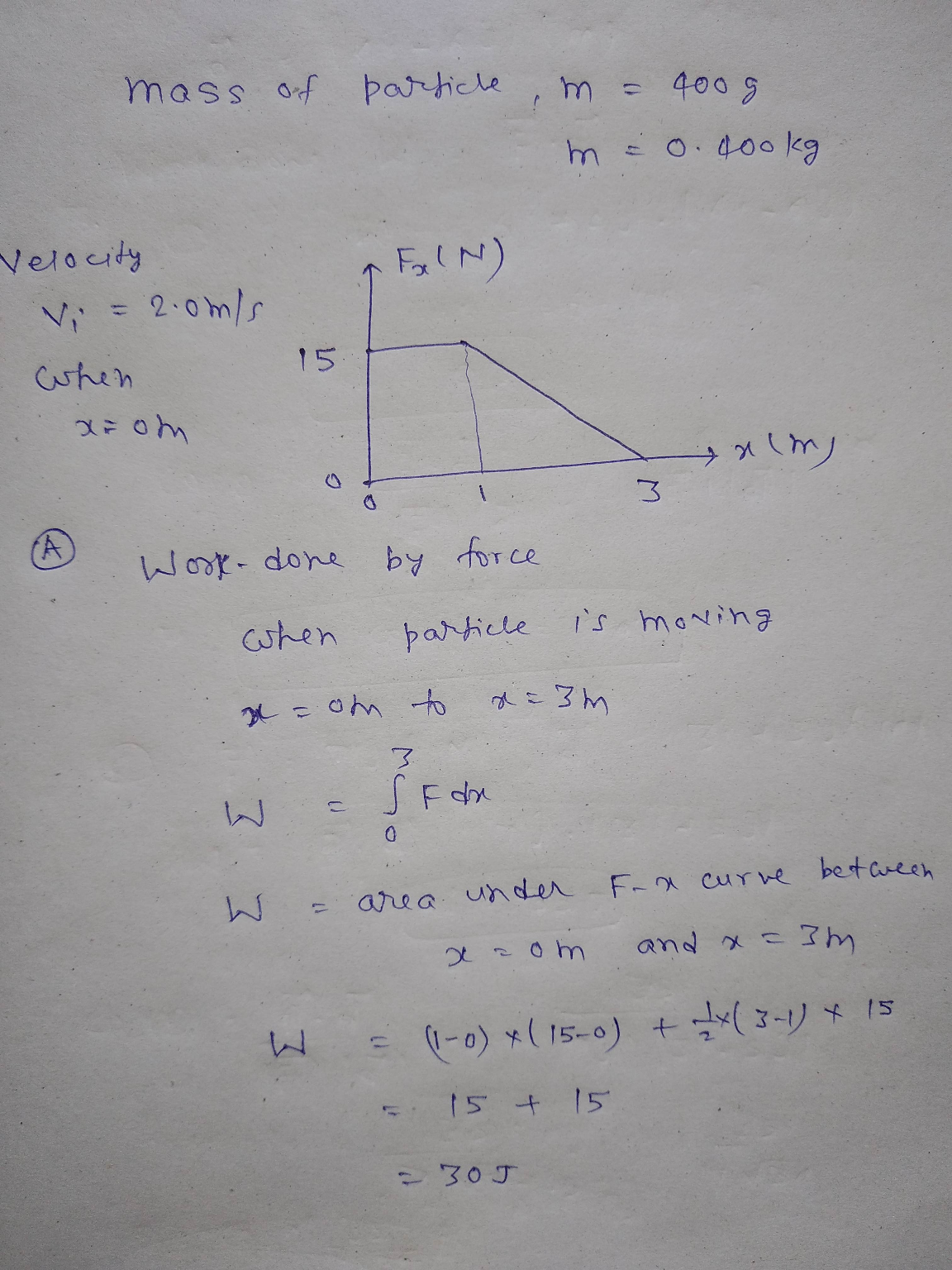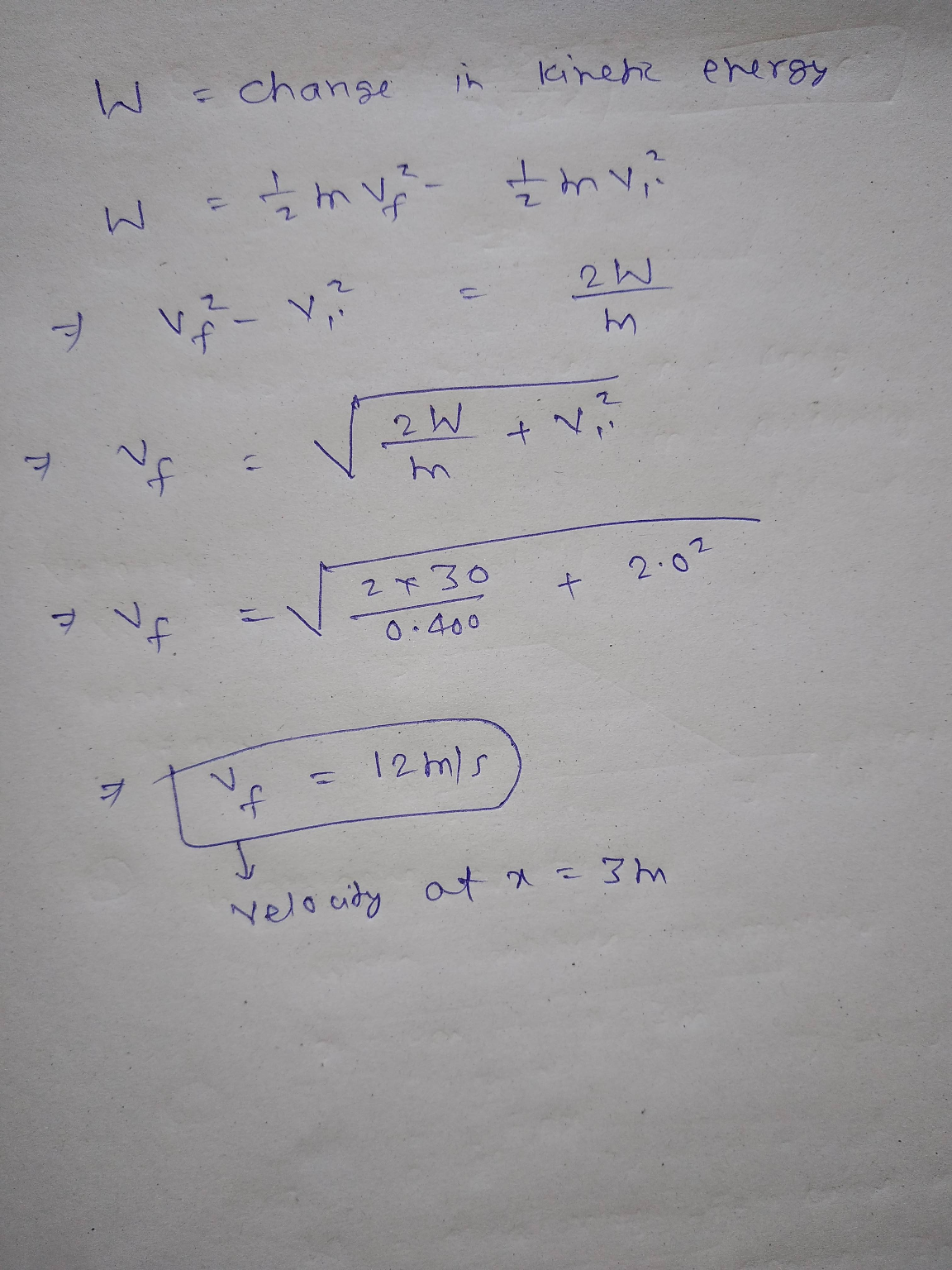#### Earn Coins

Coins can be redeemed for fabulous gifts.

Similar Homework Help Questions
• ### A 1.7 kg particle moving along the x-axis experiences the force shown in the figure(Figure 1). The particle's velocity is 5.6 m/s at x=0m.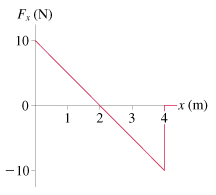A 1.7 kg particle moving along the x-axis experiences the force shown in the figure(Figure 1). The particle's velocity is 5.6 m/s at x=0m. You may want to review (Pages 214 - 218) For help with math skills, you may want to review: The Definite Integral Part A What is its velocity at 2=2 m? Part B What is its velocity at 2 = 4 m?

• ### A 1.6 kg particle moving along the x-axis experiences the force shown in the figure(Figure 1).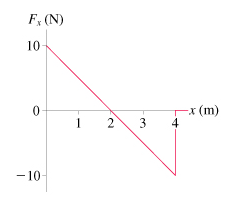A 1.6 kg particle moving along the x-axis experiences the force shown in the figure(Figure 1). The particle's velocity is 3.9 m/s at a = 0m. You may want to review (Pages 214 - 218) . For help with math skills, you may want to review: Part A What is its velocity at x = 2m? Part B What is its velocity at x = 4m? A 11-cm-long spring is attached to the ceiling. When a 1.9 kg mass is hung from it, the spring stretches...

• ### The velocity-versus-time graph is shown for a particle moving along the x-axis. Its initial position is x0 = 2.3 m at t0 = 0 s.(Figure 1)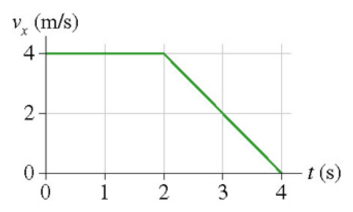The velocity-versus-time graph is shown for a particle moving along the x-axis. Its initial position is x0 = 2.3 m at t0 = 0 s.(Figure 1) You may want to review (Pages 44 - 48) . Part B What is the particle's velocity at t = 1.0 s? Part C What is the particle's acceleration at t = 1.0 s?Part DWhat is the particle's position at t =3.0s ?Express your answer to three significant figures and include the appropriate units.x=?Part EWhat is the particle's velocity...

• ### A 3.5 kg particle moving along the x-axis experiences the force shown in the figure. The...

A 3.5 kg particle moving along the x-axis experiences the force shown in the figure. The particle's velocity is 2.5 m/s at x = 0 m. What is its velocity at x = 2 m and4 m? (Indicate the direction with the sign of your answer. Assume the particle is initially traveling in the positive x-direction.)

• ### The figure(Figure 1) shows the velocity graph of a particle moving along the x-axis.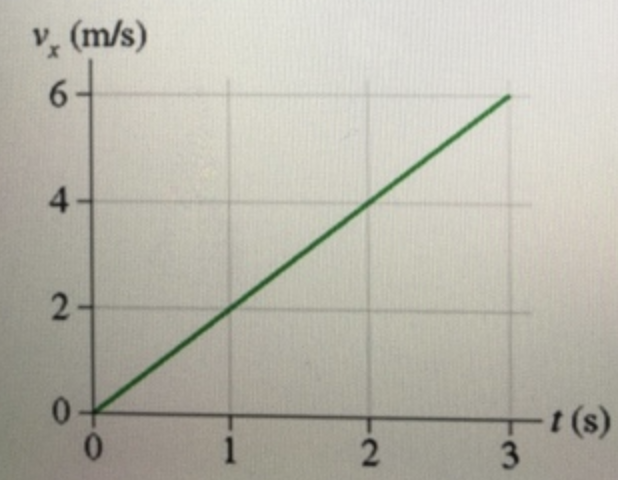The figure(Figure 1) shows the velocity graph of a particle moving along the x-axis. Its initial position is x0=2 m at t0 =0. At t=3 s, what are the particle's (a) position, (b) velocity, and (c) acceleration?Part AExpress your answer to two significant figures and include the appropriate units.Part BExpress your answer to two significant figures and include the appropriate units.Part CExpress your answer to two significant figures and include the appropriate units.

• ### The figure(Figure 1) shows the velocity graph of a particle moving along the x-axis. Its initial position is x0 = 2 m at t0 = 0. At t = 3 s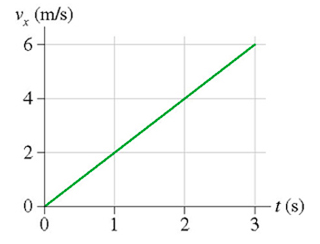The figure(Figure 1) shows the velocity graph of a particle moving along the x-axis. Its initial position is x0 = 2 m at t0 = 0. At t = 3 s , what are the particle's (a) position, (b) velocity, and (c) acceleration? Part A Express your answer to two significant figures and include the appropriate units. Part B Express your answer to two significant figures and include the appropriate units. Part C Express your answer to two significant figures and include the...

• ### (Figure 1) shows the velocity graph of a particle moving along the x-axis. Its initial position is x0=2.0m at t0=0s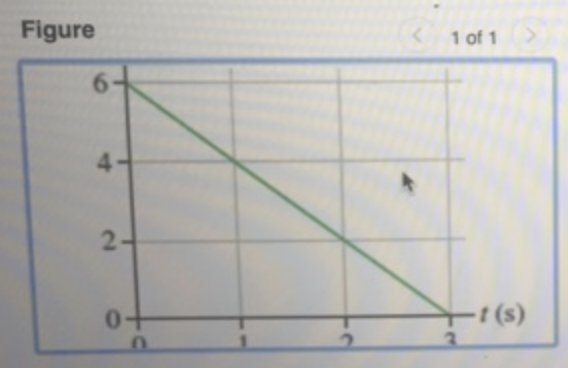(Figure 1) shows the velocity graph of a particle moving along the x-axis. Its initial position is x0=2.0 m at t0=0 sYou may want to review (Pages 44-48 ) .Part AAt t=2.0 s, what is the particle's position?Part BAt t=2.0 s, what is the particle's velocity?Part CAt t=2.0 s, what is the particle's acceleration?

• ### A 5.06kg particle moving along the x-axis experiences the force shown, where Fmax = 7.51N, Fmin...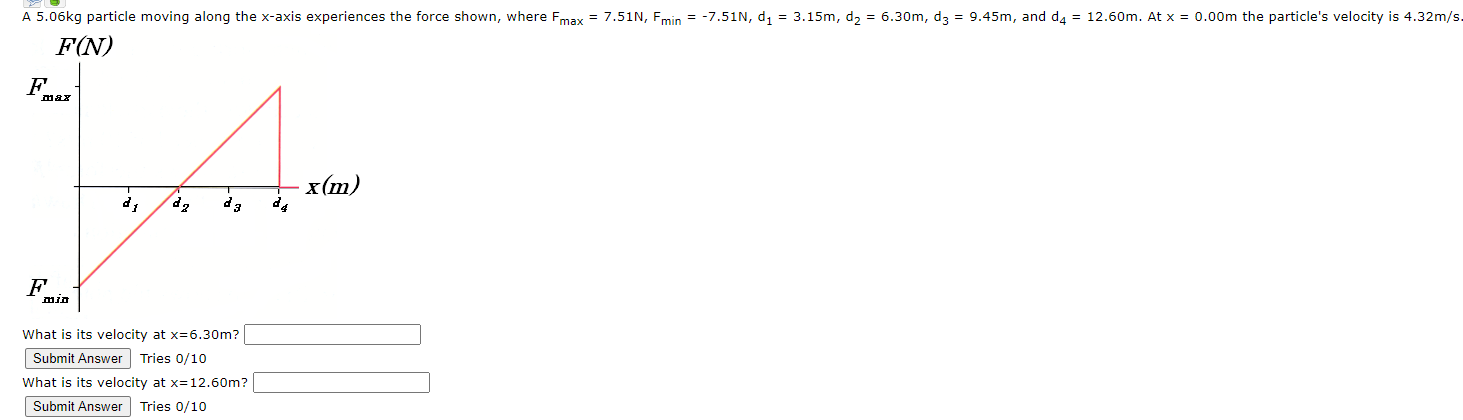A 5.06kg particle moving along the x-axis experiences the force shown, where Fmax = 7.51N, Fmin = -7.51N, d1 = 3.15m, d2 = 6.30m, dz = 9.45m, and d4 = 12.50m. At x = 0.00m the particle's velocity is 4.32m/s. FN) max 14 x(m) F MID What is its velocity at x=6.30m? Submit Answer Tries 0/10 What is its velocity at x=12.60m? Submit Answer Tries 0/10

• ### 016. A particle moving along the x-axis experiences the force shown in the figure. What is...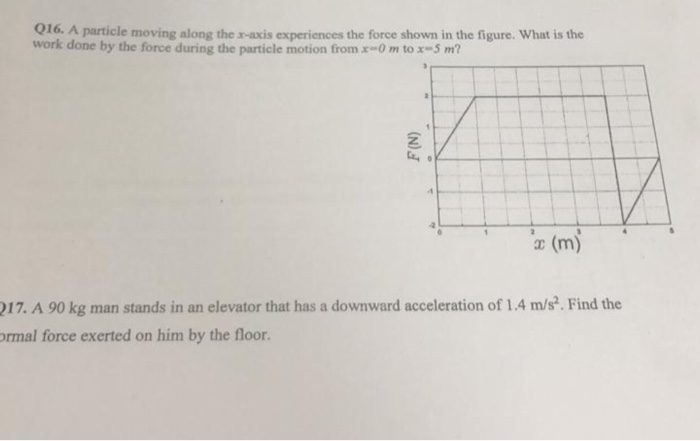016. A particle moving along the x-axis experiences the force shown in the figure. What is the work done by the force during the particle motion from x0 m tox-5 m? (N) (m) 217. A 90 kg man stands in an elevator that has a downward acceleration of 1.4 m/s. Find the ormal force exerted on him by the floor.

• ### The figure gives the acceleration a versus timet for a particle moving along an x...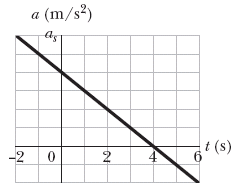The figure gives the acceleration a versus timet for a particle moving along an x axis. Thea-axis scale is set by as = 16.0 m/s2. At t = -2.0 s, the particle's velocity is 7.00 m/s. What is its velocity at t = 6.0 s?